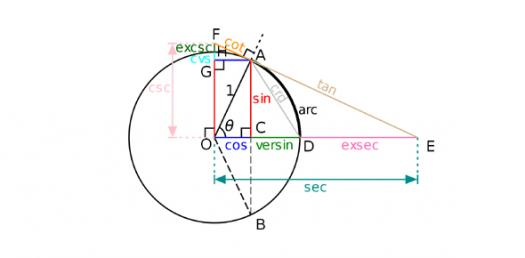# Do You Know Your Math? Trigonometry Quiz

10 Questions | Attempts: 79995SettingsTrigonometry is the study of the relationship between lengths and angles of triangles. We covered a lot of trigonometry problems I the past few weeks. Do you think you understood them all? Test your knowledge by trying to answer the quiz below. All the best and remember to keep practicing!

• 1.
What is the correct formula to find the Pythagorean Theorem?
• A.

A2+b2=c2

• B.

B2+c2=a2

• C.

A2+c2=b2

• 2.
Who created the Pythagorean Theorem?
• A.

• B.

My Mom

• C.

Pythagoras

• D.

No One

• 3.
What are the legs of a right triangle?
• A.

A,b

• B.

A,c

• C.

B,c

• 4.
What side is the hypotenuse of a right triangle?
• A.

A

• B.

B

• C.

C

• D.

G

• 5.
What is the longest side of a right triangle called?
• A.

Leg g

• B.

Leg b

• C.

Leg a

• D.

Hypotenuse

• 6.
Find the Hypotenuse if a=12 and b=16
• A.

C=93

• B.

C=42

• C.

A=20

• D.

C=20

• 7.
Find the Hypotenuse if a=5 and b=7.
• A.

C=8.60

• B.

C=19.58

• C.

B=39.84

• D.

G=47.29

• 8.
Find the length of the Leg if a=4 and c=10
• A.

G=94.58

• B.

C=37.90

• C.

B=9.17

• D.

A=Nothing

• 9.
Find the length of the leg and round to the nearest hundredth if c=10 and b=7
• A.

A=7.14

• B.

A=92.38

• C.

G=73.73

• 10.
Find the length of the missing side. if a=4 and c=5.
• A.

1

• B.

3

• C.

6.4

• D.

9

## Related TopicsBack to top
×

Wait!
Here's an interesting quiz for you.